# 十五、立体视觉和 3D 重建

• 什么是立体对应
• 什么是对极几何
• 什么是深度图
• 如何提取 3D 信息
• 如何构建和可视化给定场景的 3D 地图

# 什么是立体对应？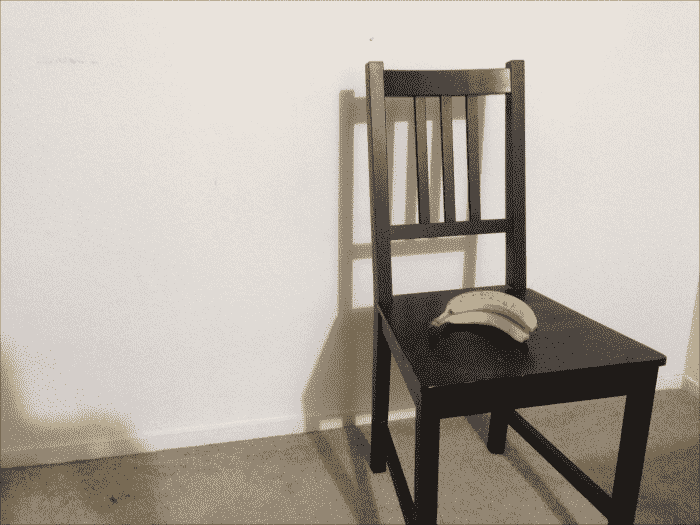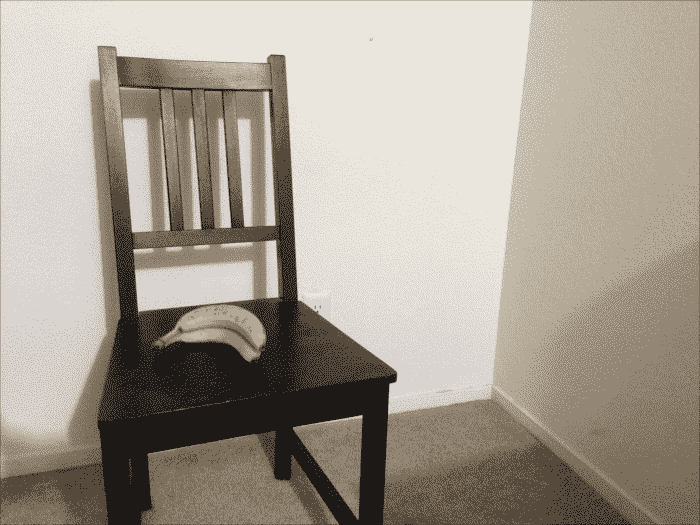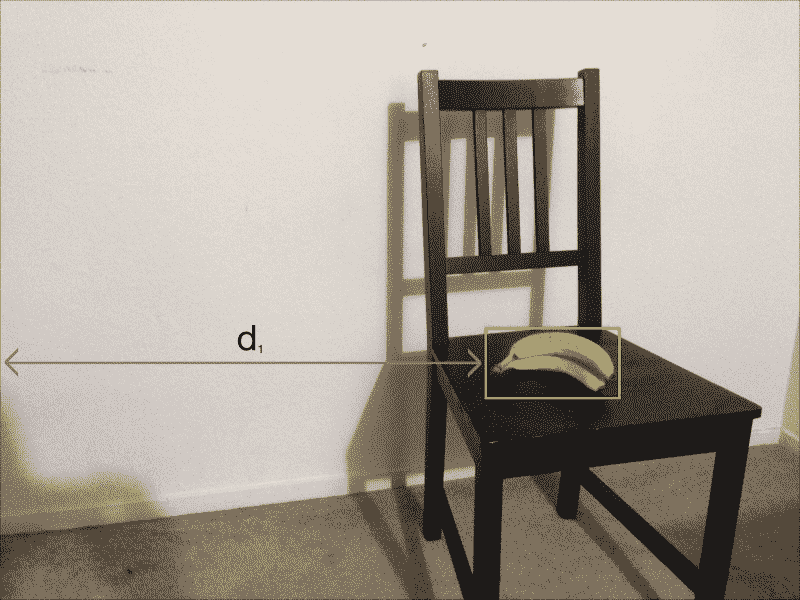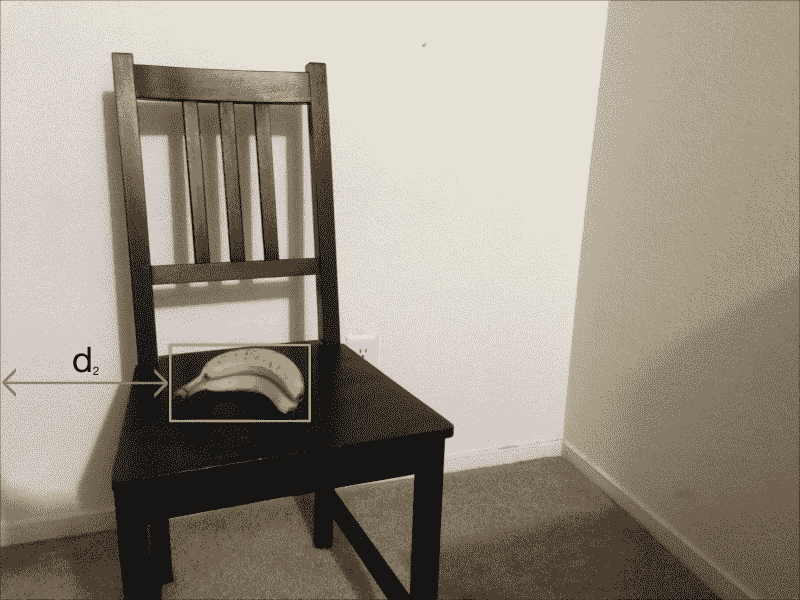d1d2之间的差异大。 现在，让盒子靠近相机：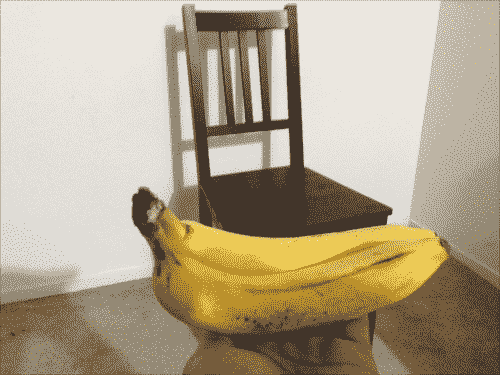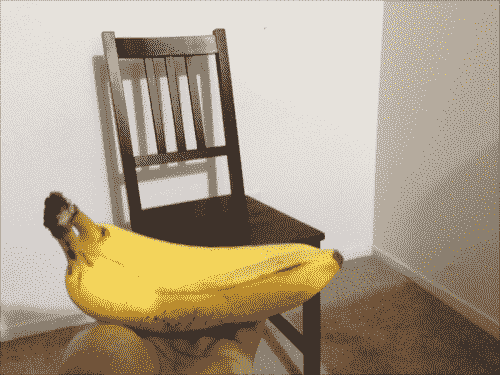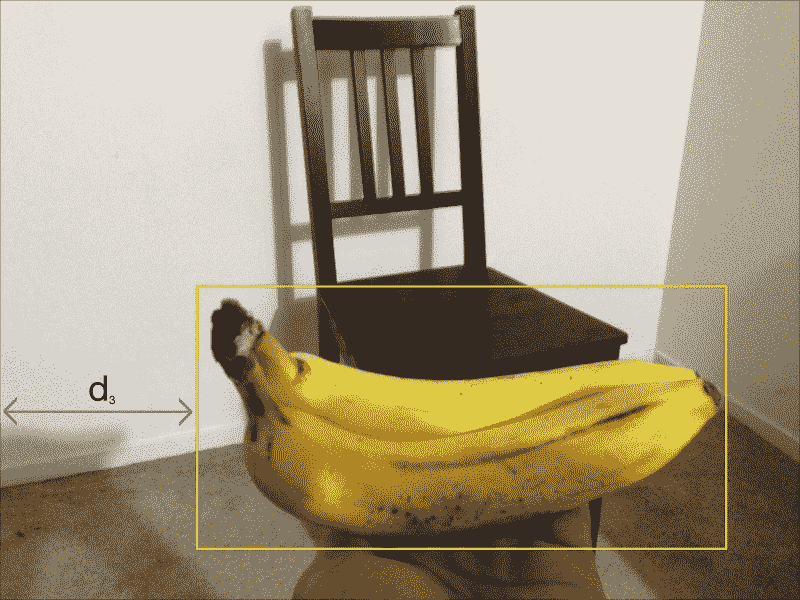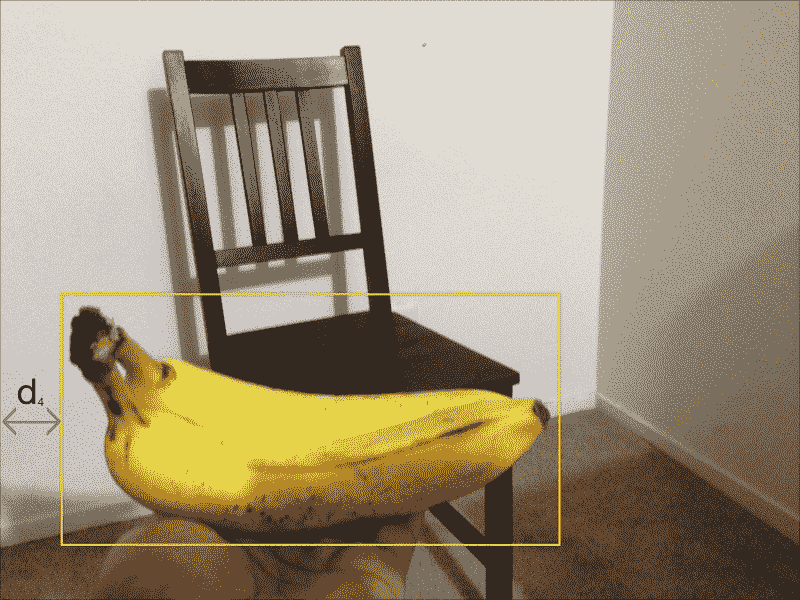d3d4之间的差异小。 可以说d1d2之间的绝对差大于d3d4之间的绝对差。 即使摄像机移动了相同的量，初始位置和最终位置之间的视在距离之间也存在很大差异。 发生这种情况是因为我们可以使物体靠近相机； 当您从不同角度拍摄两个图像时，视在运动会减少。 这就是立体对应的概念：我们捕获两个图像，并使用此知识从给定场景中提取深度信息。

# 什么是对极几何？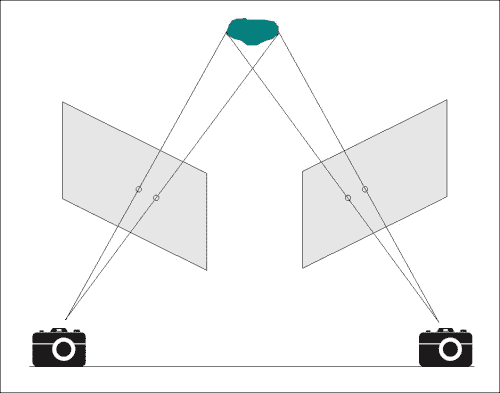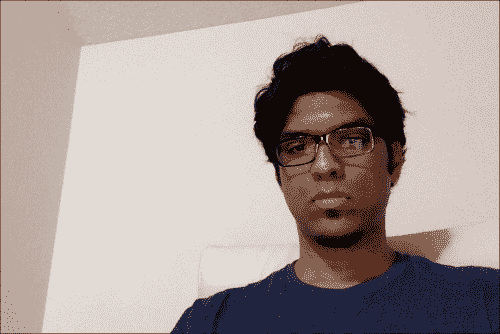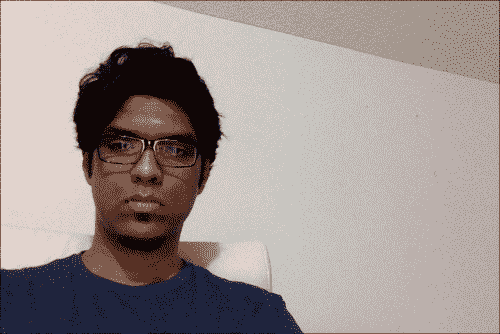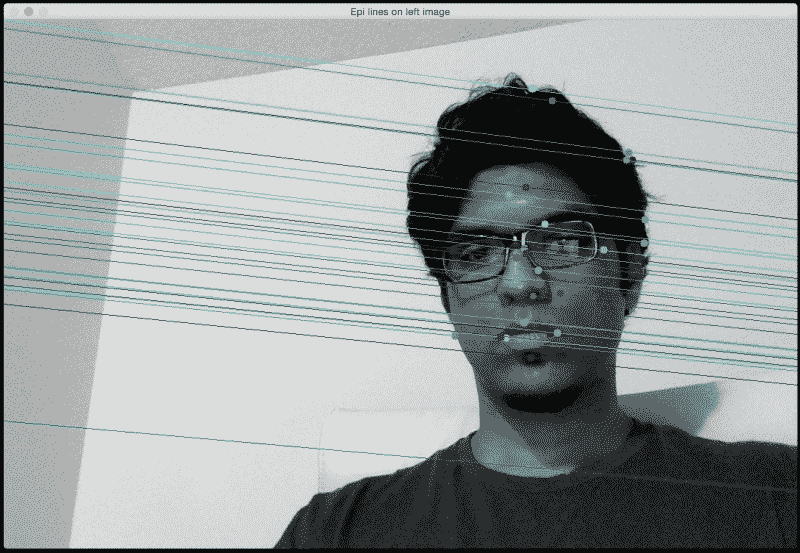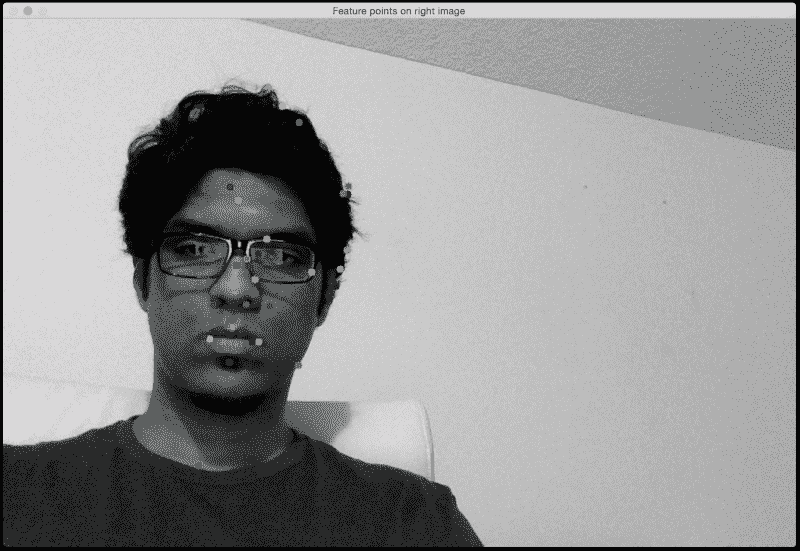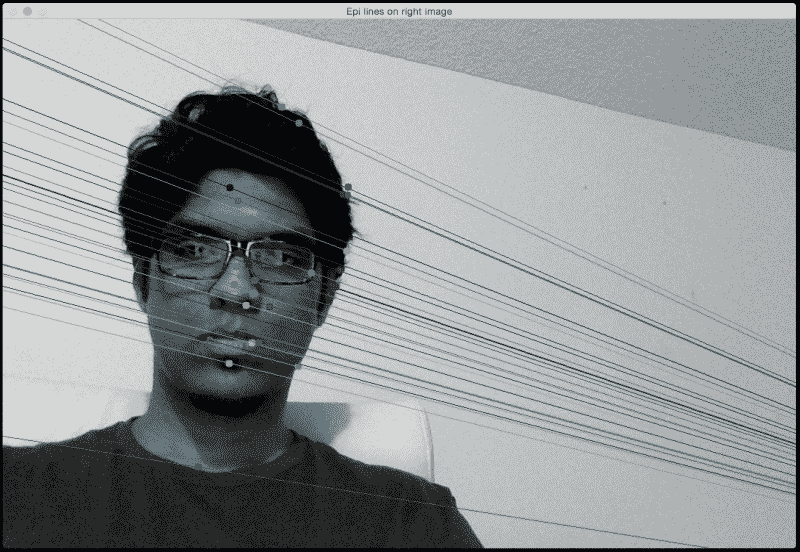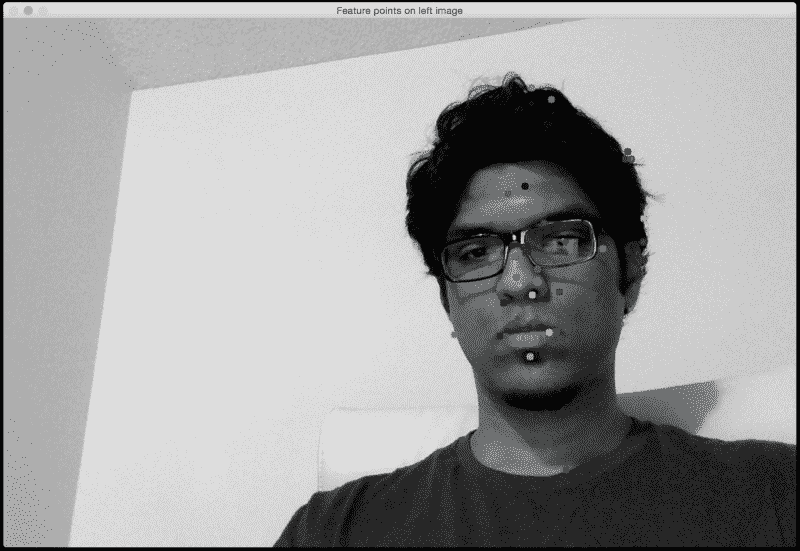import argparse

import cv2
import numpy as np

def build_arg_parser():
parser = argparse.ArgumentParser(description='Find fundamental matrix \
using the two input stereo images and draw epipolar lines')
help="Image captured from the left view")
help="Image captured from the right view")
required=True, help="Feature extractor that will be used; can be either 'sift' or 'surf'")
return parser

def draw_lines(img_left, img_right, lines, pts_left, pts_right):
h,w = img_left.shape
img_left = cv2.cvtColor(img_left, cv2.COLOR_GRAY2BGR)
img_right = cv2.cvtColor(img_right, cv2.COLOR_GRAY2BGR)

for line, pt_left, pt_right in zip(lines, pts_left, pts_right):
x_start,y_start = map(int, [0, -line/line ])
x_end,y_end = map(int, [w, -(line+line*w)/line ])
color = tuple(np.random.randint(0,255,2).tolist())
cv2.line(img_left, (x_start,y_start), (x_end,y_end), color,1)
cv2.circle(img_left, tuple(pt_left), 5, color, -1)
cv2.circle(img_right, tuple(pt_right), 5, color, -1)

return img_left, img_right

def get_descriptors(gray_image, feature_type):
if feature_type == 'surf':
feature_extractor = cv2.SURF()

elif feature_type == 'sift':
feature_extractor = cv2.SIFT()

else:
raise TypeError("Invalid feature type; should be either 'surf' or 'sift'")

keypoints, descriptors = feature_extractor.detectAndCompute(gray_image, None)
return keypoints, descriptors

if __name__=='__main__':
args = build_arg_parser().parse_args()
img_left = cv2.imread(args.img_left,0)  # left image
img_right = cv2.imread(args.img_right,0)  # right image
feature_type = args.feature_type

if feature_type not in ['sift', 'surf']:
raise TypeError("Invalid feature type; has to be either 'sift' or 'surf'")

scaling_factor = 1.0
img_left = cv2.resize(img_left, None, fx=scaling_factor,
fy=scaling_factor, interpolation=cv2.INTER_AREA)
img_right = cv2.resize(img_right, None, fx=scaling_factor,
fy=scaling_factor, interpolation=cv2.INTER_AREA)

kps_left, des_left = get_descriptors(img_left, feature_type)
kps_right, des_right = get_descriptors(img_right, feature_type)

# FLANN parameters
FLANN_INDEX_KDTREE = 0
index_params = dict(algorithm = FLANN_INDEX_KDTREE, trees = 5)
search_params = dict(checks=50)

# Get the matches based on the descriptors
flann = cv2.FlannBasedMatcher(index_params, search_params)
matches = flann.knnMatch(des_left, des_right, k=2)

pts_left_image = []
pts_right_image = []

# ratio test to retain only the good matches
for i,(m,n) in enumerate(matches):
if m.distance < 0.7*n.distance:
pts_left_image.append(kps_left[m.queryIdx].pt)
pts_right_image.append(kps_right[m.trainIdx].pt)

pts_left_image = np.float32(pts_left_image)
pts_right_image = np.float32(pts_right_image)
F, mask = cv2.findFundamentalMat(pts_left_image, pts_right_image, cv2.FM_LMEDS)

# Selecting only the inliers

# Drawing the lines on left image and the corresponding feature points on the right image
lines1 = cv2.computeCorrespondEpilines (pts_right_image.reshape(-1,1,2), 2, F)
lines1 = lines1.reshape(-1,3)
img_left_lines, img_right_pts = draw_lines(img_left, img_right, lines1, pts_left_image, pts_right_image)

# Drawing the lines on right image and the corresponding feature points on the left image
lines2 = cv2.computeCorrespondEpilines (pts_left_image.reshape(-1,1,2), 1,F)
lines2 = lines2.reshape(-1,3)
img_right_lines, img_left_pts = draw_lines(img_right, img_left, lines2, pts_right_image, pts_left_image)

cv2.imshow('Epi lines on left image', img_left_lines)
cv2.imshow('Feature points on right image', img_right_pts)
cv2.imshow('Epi lines on right image', img_right_lines)
cv2.imshow('Feature points on left image', img_left_pts)
cv2.waitKey()
cv2.destroyAllWindows()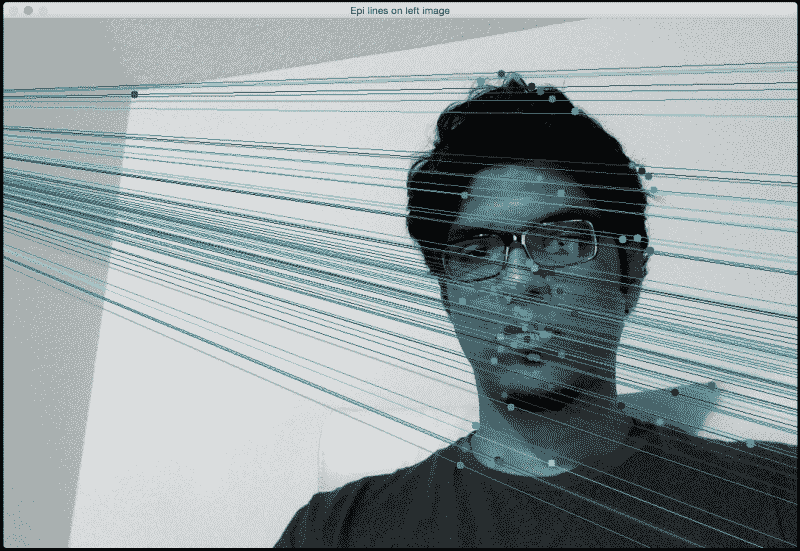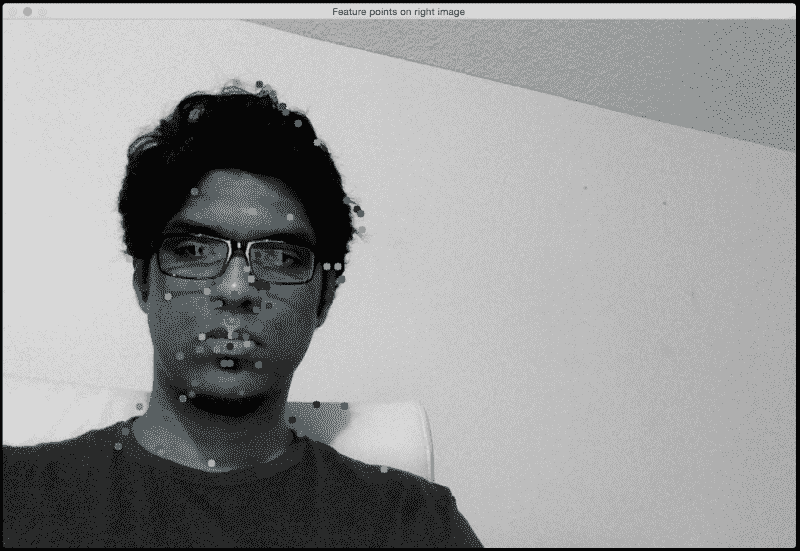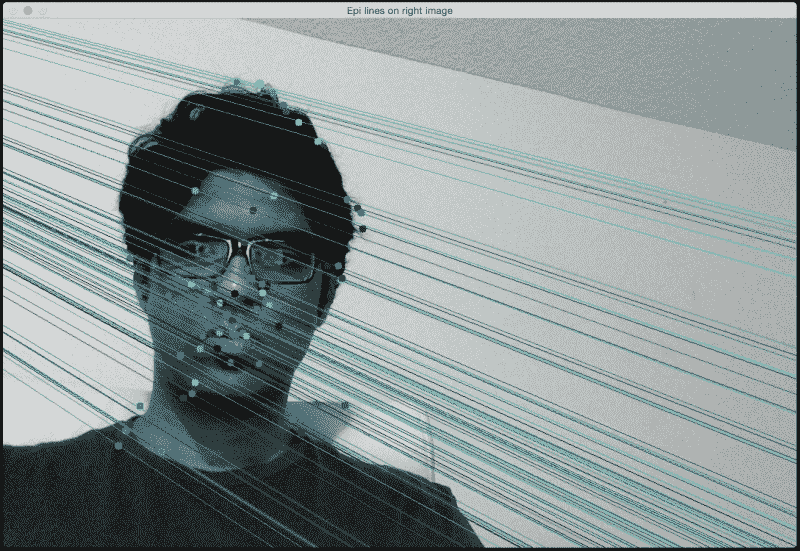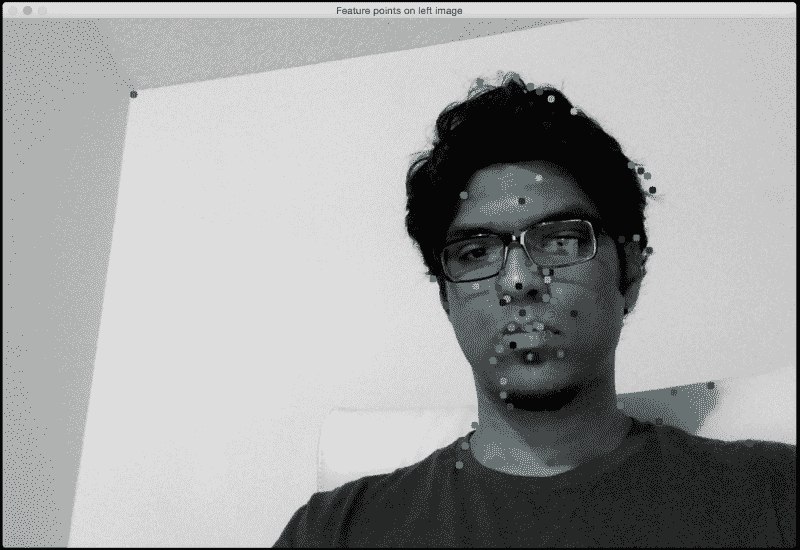## 与 SIFT 相比，SURF 为何不同？

SURF 检测到一组不同的特征点，因此相应的对极线也不同。 正如您在图像中看到的那样，当我们使用 SURF 时会检测到更多特征点。 由于我们比以前拥有更多的信息，因此相应的对极线也将相应更改。

# 建立 3D 地图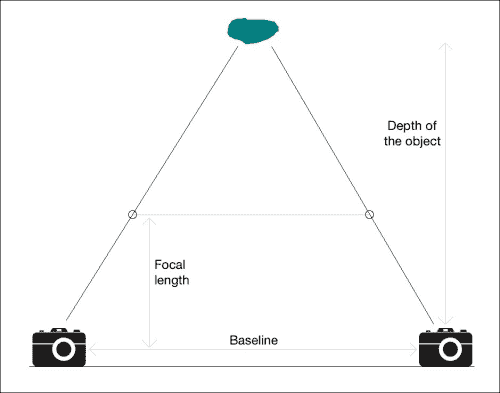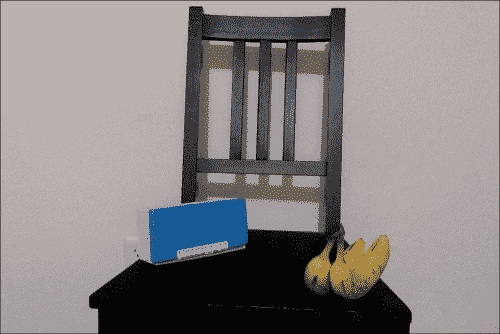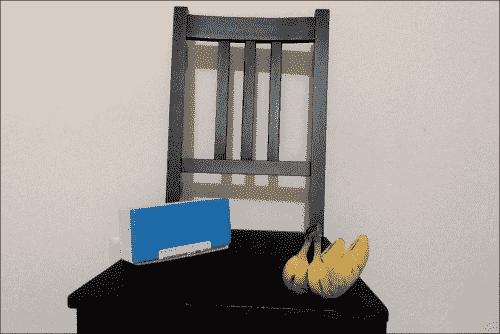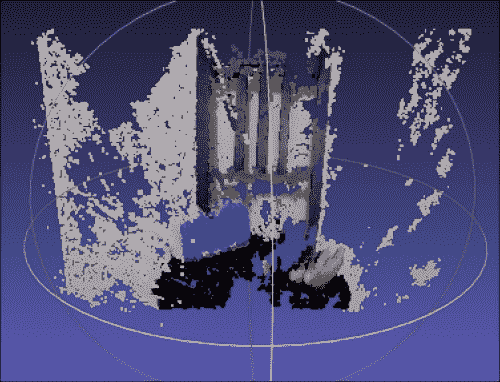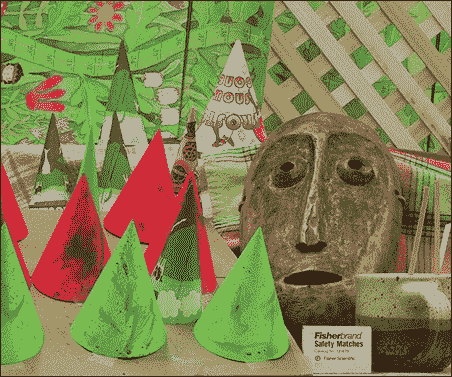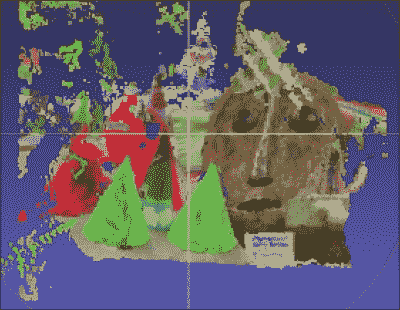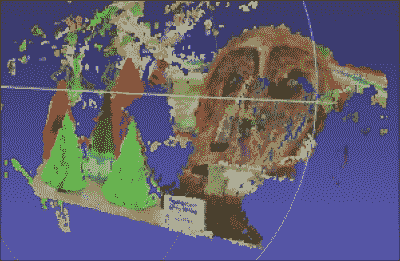import argparse

import cv2
import numpy as np

def build_arg_parser():
parser = argparse.ArgumentParser(description='Reconstruct the 3D map from \
the two input stereo images. Output will be saved in \'output.ply\'')
help="Input image captured from the left")
help="Input image captured from the right")
help="Output filename (without the extension) where the point cloud will be saved")
return parser

def create_output(vertices, colors, filename):
colors = colors.reshape(-1, 3)
vertices = np.hstack([vertices.reshape(-1,3), colors])

format ascii 1.0
element vertex %(vert_num)d
property float x
property float y
property float z
property uchar red
property uchar green
property uchar blue
'''

with open(filename, 'w') as f:
np.savetxt(f, vertices, '%f %f %f %d %d %d')

if __name__ == '__main__':
args = build_arg_parser().parse_args()
output_file = args.output_file + '.ply'

if image_left.shape != image_right.shape or \
image_left.shape != image_right.shape:
raise TypeError("Input images must be of the same size")

# downscale images for faster processing
image_left = cv2.pyrDown(image_left)
image_right = cv2.pyrDown(image_right)

# disparity range is tuned for 'aloe' image pair
win_size = 1
min_disp = 16
max_disp = min_disp * 9
num_disp = max_disp - min_disp   # Needs to be divisible by 16
stereo = cv2.StereoSGBM(minDisparity = min_disp,
numDisparities = num_disp,
uniquenessRatio = 10,
speckleWindowSize = 100,
speckleRange = 32,
disp12MaxDiff = 1,
P1 = 83win_size**2,
P2 = 323win_size**2,
fullDP = True
)

print "\nComputing the disparity map ..."
disparity_map = stereo.compute(image_left, image_right).astype(np.float32) / 16.0

print "\nGenerating the 3D map ..."
h, w = image_left.shape[:2]
focal_length = 0.8*w

# Perspective transformation matrix
Q = np.float32([[1, 0, 0, -w/2.0],
[0,-1, 0,  h/2.0],
[0, 0, 0, -focal_length],
[0, 0, 1, 0]])

points_3D = cv2.reprojectImageTo3D(disparity_map, Q)
colors = cv2.cvtColor(image_left, cv2.COLOR_BGR2RGB)

print "\nCreating the output file ...\n"
create_output(output_points, output_colors, output_file)